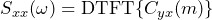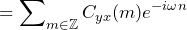# Cross-Spectral Density (CSD)

June 2, 2021

The cross-spectral density (CSD) function is the discrete-time Fourier transform of the cross-correlation function Cyx(m). It is defined as:

(1)(2)The CSD expresses how correlated a signal x is in relation to another signal y. The CSD is nearly the same as the auto spectral density (ASD), except the signal may be compared to another signal.

The ASD of x(n) is a special case of the CSD of x(n) and y(n), where y(n)=x(n). That is, Rxx(m)=Cxx(m).

### Examples

• A pair of signals with CSD=0 indicates they are very uncorrelated.
• If the CSD is flat but non-zero, then y and x are uncorrelated but are either the same signal or almost the same signal.
• If the CSD has a main lobe, then the pair of signals has some degree of correlation. In general, the wider the main lobe, the more uncorrelated the pair. Likewise, the narrower the main lobe, the more correlated the pair.
• A CSD with a spike at some frequency (F) in Hertz indicates the pair of signals is periodically correlated every 1/F seconds. This may be due to a resonant frequency at F.
• If the CSD is nearly flat, then the samples are pretty much uncorrelated.
• A CSD with a single spike at 0Hz is likely caused by the presence of a large DC offset in both signals.
• A CSD with a medium width main lobe indicates that samples in one signal are correlated with other samples in the other located close in time, but that the correlation diminishes with separation in time.

### CSD and Spectral Power

The CSD and power spectral density (PSD) are closely related. In fact, the PSD is a special case of CSD. If two signals x and y analyzed by the CSD are the same (x=y), then the CSD is the PSD. And, just as with PSD, the true CSD is unknowable but can be estimated.